# The conv function and implementation tradeoffs

A friend from my grad school days (back in the previous millenium) is an electrical engineering professor. Students in his class recently asked him why the conv function is not implemented using FFTs.

I'm not on the team responsible for conv, but I wrote back with my thoughts, and I thought I would share them here as well.

Let's review the basics. Using the typical convolution formula to compute the one-dimensional convolution of a P-element sequence A with Q-element sequence B has a computational complexity of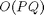. However, the discrete Fourier transform (DFT) can be used to implement convolution as follows:

1. Compute the L-point DFT of A, where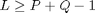.

2. Compute the L-point DFT of B.

3. Multiply the two DFTs.

4. Compute the inverse DFT to get the convolution.

Here's a simple MATLAB function for computing convolution using the Fast Fourier Transform (FFT), which is simply a fast algorithm for computing the DFT.

type conv_fft
function c = conv_fft(a, b)

P = numel(a);
Q = numel(b);
L = P + Q - 1;
K = 2^nextpow2(L);

c = ifft(fft(a, K) .* fft(b, K));
c = c(1:L);



Note that the code uses the next power-of-two greater than or equal to L, although this is not strictly necessary. The fft function operates in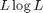time whether or not L is a power of two.

The overall computational complexity of these steps is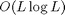. For P and Q sufficiently large, then, using the DFT to implement convolution is a computational win.

So why don't we do it?

There are several technical factors. Let's look at speed, exact computation, and memory.

Speed

One factor is that DFT-based computation is not always faster. Let's do an experiment where we compute the convolution of a 1000-element sequence with another sequence of varying length.

x = rand(1, 1000);
nn = 25:25:1000;

t_normal = zeros(size(nn));
t_fft = zeros(size(nn));

for k = 1:numel(nn)
n = nn(k);
y = rand(1, n);
t_normal(k) = timeit(@() conv(x, y));
t_fft(k) = timeit(@() conv_fft(x, y));
end
plot(nn, t_normal, nn, t_fft)
legend({'Normal computation', 'FFT-based computation'})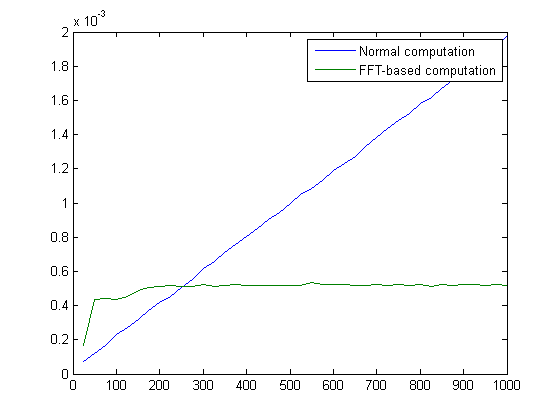For sequences y shorter than a certain length, called the cross-over point, it's quicker to use the normal computation.

Exact computation

A second consideration is whether the computation is subject to floating-point round-off errors and to what degree. There are applications, for example, where the convolution of integer-valued sequences is computed. For such applications, a user would reasonably expect the output sequence to be integer-valued as well.

To illustrate, here's a simple function that computes n-th order binomial coefficients using convolution:

type binom
function c = binom(n)

c = 1;
for k = 1:n
c = conv(c, [1 1]);
end


binom(7)
ans =

1     7    21    35    35    21     7     1



I wrote a variation called binom_fft that is the same as binom except that it calls conv_fft.

format long
binom_fft(7)
ans =

Columns 1 through 4

1.000000000000000   6.999999999999998  21.000000000000000  35.000000000000000

Columns 5 through 8

35.000000000000000  21.000000000000000   7.000000000000000   0.999999999999996



Whoops! What's going on? The answer is that the FFT-based implementation of convolution is subject to floating-point round-off error.

I imagine that most MATLAB users would consider the output of binom_fft to be wrong.

Memory

The last technical consideration I want to mention is memory. Because of the padding and complex arithmetic involved in the FFT computations, the FFT-based convolution implementation requires a lot more memory than the normal computation. This may not often be a problem for one-dimensional computations, but it can be a big deal for multidimensional computations.

Final thoughts

The technical considerations listed above can all be solved in principle. The implementation could switch to using the normal method for short or integer-valued sequences, for example. And there are FFT-based techniques such as overlap-and-add to reduce the memory load.

But the problem can get quite complicated. Testing floating-point values to see if they are integers, for example, can be slow. Also, I suspect (but have not checked) that a multithreaded implemention of the normal computation will take advantage of multiple cores better than a multithreaded FFT-based method. That would change the cross-over point between the two methods. And the exact cross-over point will vary from computer to computer, making it likely that our implementation would be somewhat slower for some people for some problems.

Overall, my inclination would be to provide FFT-based convolution as a separate function rather than reimplementing conv. And that's what we've done. See the function fftfilt in the Signal Processing Toolbox.

Do you disagree with this approach? Post your comment.

Published with MATLAB® 7.9

|